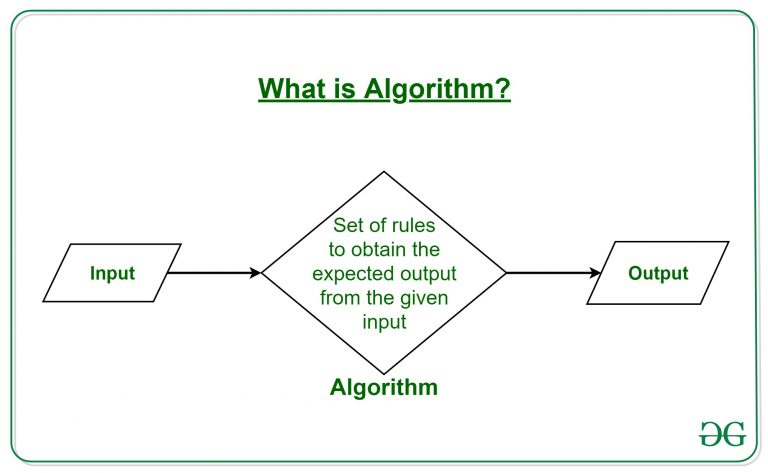#### What is Algorithm?

Basics of an Algorithm

The term Algorithm refers to the "set of unambiguous instructions to be followed for solving a given problem statement". Thus, we can say the step-by-step definition of a problem or the neccesary work to be done in order to get the desired output. It can be thought as a recipe for a delicious and tasty dish.Picture from GeeksforGeeks

Algorithms play a major role in the solution of various problem statements in the field of Mathematics and Computer Science. Let us take an example to understand the content in more detail. Suppose we have a problem which says, "Print all Odd Numbers from 1 to 100"

Code can be written as follows:
``` ```
// Code in C++
#include
using namespace std;
void main(){
int i=0 ;
for(i=0; i<=100; i++)
{
if(i%2==1)  //or (i%2 != 0)
cout<< i<< endl;
}
}```
```

Now we write steps for the same question whose code is given above.
Step 1: Assign a variable of type integer 'i'
Step 2: Set i=0, and write condition of for loop(i++)
Step 3: Check if i <= 100 before for loop
Step 4: Check if i%2==1 i.e. odd number then print i
Step 5: Repeat steps 1 to 4 untill i > 100
Step 6: End
The above steps represent the algorithm for the given question. These steps define the working of the algorithm in an orderly manner.The above diagram is the flow chart for printing odd numbers from 1 to 100. Please emphasize on the arrows.

There can be many solutions to the same problem, for example, the above program can be written using a while loop, or manually printing each number(not a good practice) or switch case(too tedious). Thus we can conclude that there are multiple or more than one way to solve a problem. So there should be some methods to examine which algorithm is good or bad. There is no such good or bad algorithm, it all depends on its efficiency.

The efficiency tells how much time or/and space the algorithm takes while executing. Calculation of these two factors requires the introduction of two new terms, Time complexity, and Space complexity.

##### Time complexity:
The time calculation to execute the algorithm chosen
##### Space complexity:
The space occupied for running the algorithm chosen

The detailed analysis of the algorithm can be classified under a different domain, Analysis of Algorithms.

Though we are stoping here for this blog, there is lot more to explore on Algorithms.